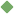quantity 1. [ noun ] how much there is of something that you can measure Synonyms: amount measure quantum Related terms: abstraction time_period probability volume value time_interval point fundamental_measure volume proof relative_quantity indefinite_quantity playing_period cordage system_of_measurement quantum octane_number time_unit linear_measure magnetisation magnetic_flux definite_quantity measure total standard nonstandard To share this definition press "text" (Facebook, Twitter) or "link" (blog, mail) then paste text link 2. [ noun ] an adequate or large amount Examples: "he had a quantity of ammunition" Related terms: amount abundance sufficiency sufficient insufficient abundant scarce 3. [ noun ] (mathematics) something that has a magnitude and can be represented in mathematical expressions by a constant or a variable Related terms: concept difference product sum constant term variable binomial operand quotient proportional numerical_quantity quantum quantum parameter quantify Similar spelling: quintet